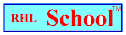Name_______________________________________________Date_________________________
Mathematics Problem Solving
Volume 5, Number 8, October 25, 1999
www.rhlschool.com

### Rounding Riddles

1. I am a number. If you round the number of days in October to the nearest ten and round the number of days in February to the nearest ten, I am half of the product of those two numbers. What number am I?

2. I am an amount of U.S. money. I am the cost of five \$ .88 hamburgers rounded to the nearest dollar. How much money am I?

3. I am a number. I am the difference between 800 rounded to the nearest 10 and 800 rounded to the nearest 100. How much am I?

4. I am a number. I am the missing number from each of two of the equations below. I am also the sum of 237 and 240 rounded to the nearest 10. Which two equations do I complete?

a. 1,782 - 800 =
b. 8 X 60 =
c. 480 + 20 =
d. 5,322 - 4,842 =

5. I am a number. I am the smallest number that can become 500 when it’s rounded to the nearest 10. What number am I?

6. I am a number. I am the largest whole number that must equal two thousand when rounded to the nearest thousand. What number am I?

7. I am a number. I am the sum of 1,270 rounded to the nearest 10, 1,270 rounded to the nearest 100, and 1,270 rounded to the nearest 1,000. What number am I?

rhlschool.com

RHL School - Free Worksheets and MoreCopyright 1999 RHL

RHL School Home